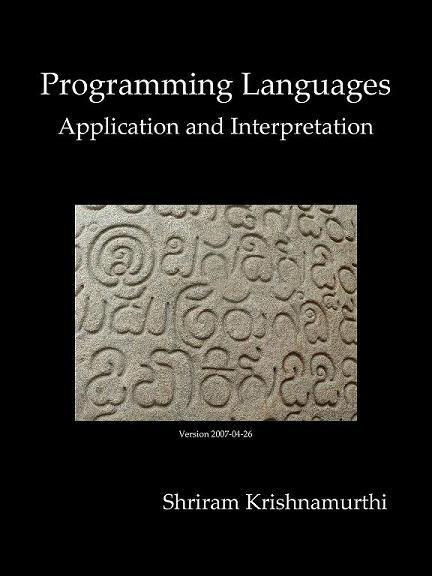GitHub：PLAI-cn

GitBook：PLAI-cn

## 15.1 静态类型规则

``````(define (f [n : number]) : number
(+ n 3))

(f "x")
``````

``````(define (f n)
(+ n 3))

(f "x")
``````

``````(define f n
(+ n 3))
``````

## 15.2.1 简单的类型检查器

``````(define-type TyExprC
[numC (n : number)]
[idC (s : symbol)]
[appC (fun : TyExprC) (arg : TyExprC)]
[plusC (l : TyExprC) (r : TyExprC)]
[multC (l : TyExprC) (r : TyExprC)]
[lamC (arg : symbol) (argT : Type) (retT : Type) (body : TyExprC)])
``````

``````(define-type Value
[numV (n : number)]
[closV (arg : symbol) (body : TyExprC) (env : Env)])
``````

``````(define-type Type
[numT]
[funT (arg : Type) (ret : Type)])
``````

• `+`的参数不是数，即不是`numT`
• `*`的参数不是数。
• 函数调用时函数位置的表达式不是函数，即不是`funT`

• 函数调用时实参的类型和函数形参的类型不一致。

``````<tc-take-1> ::=  ;;类型检查，第一次尝试

(define (tc [expr : TyExprC] [tenv : TyEnv]) : boolean
(type-case TyExprC expr
<tc-take-1-numC-case>
<tc-take-1-idC-case>
<tc-take-1-appC-case>))
``````

``````<tc-take-1-numC-case> ::=

[numC (n) true]
``````

``````<tc-take-1-idC-case> ::=

[idC (n) (if (lookup n tenv)
true
(error 'tc "not a bound identifier"))]  ;不是绑定标识符
``````

``````<tc-take-1-appC-case> ::=

[appC (f a) (let ([ft (tc f tenv)])
...)]
``````

``````<tc> ::=

(define (tc [expr : TyExprC] [tenv : TyEnv]) : Type
(type-case TyExprC expr
<tc-numC-case>
<tc-idC-case>
<tc-plusC-case>
<tc-multC-case>
<tc-appC-case>
<tc-lamC-case>))
``````

``````<tc-numC-case> ::=

[numC (n) (numT)]
``````

``````<tc-idC-case> ::=

[idC (n) (lookup n tenv)]
``````

``````<tc-plusC-case> ::=

[plusC (l r) (let ([lt (tc l tenv)]
[rt (tc r tenv)])
(if (and (equal? lt (numT))
(equal? rt (numT)))
(numT)
(error 'tc "+ not both numbers")))] ;+不都是数
``````

``````<tc-multC-case> ::=

[multC (l r) (let ([lt (tc l tenv)]
[rt (tc r tenv)])
(if (and (equal? lt (numT))
(equal? rt (numT)))
(numT)
(error 'tc "* not both numbers")))] ;*不都是数
``````

``````<tc-appC-case> ::=

[appC (f a) (let ([ft (tc f tenv)]
[at (tc a tenv)])
(cond
[(not (funT? ft))
(error 'tc "not a function")] ;不是函数
[(not (equal? (funT-arg ft) at))
(error 'tc "app arg mismatch")] ;app参数不匹配
[else (funT-ret ft)]))]
``````

``````<tc-lamC-case> ::=

[lamC (a argT retT b)
(if (equal? (tc b (extend-ty-env (bind a argT) tenv)) retT)
(funT argT retT)
(error 'tc "lam type mismatch"))] ;λ类型不匹配
``````

## 15.2.2 条件语句的类型检查

1. 条件表达式的类型应该是什么？某些语言中它必须计算得到布尔值，这种情况下需要为我们的语言添加布尔值类型（这可能是个好主意）。其它语言中，它可以是任意值，某些值被认为是“真值”，其它的则被视为“假值”。
2. `then-``else-`两个分支之间的关系应该是什么呢？一些语言中它们的类型必须相同，因此整个 if 表达式有一个确定无歧义的类型。其它语言中，两个分支可以有不同的类型，这极大地改变了静态类型语言的设计和它的类型检查器，而且也改变了编程语言本身的性质。

## 15.2.3.1 递归的类型，初次尝试

``````((lambda (x) (x x))
(lambda (x) (x x)))
``````

## 15.2.3.2 程序终止

JavaScript 中的回调；甚至编译器或链接器。每种情况下，我们都有一个不成文的期望，即这些程序最终会终止。而现在我们有一个语言能保证这点——且这点是不可测试的。

## 15.2.3.3 静态类型的递归

``````(rec (Σ num (n num)
(if0 n
0
(n + (Σ (n + -1))))) ;译注，原文如此，+应前置
(Σ 10))
``````

``````<tc-lamC-case> ::=

[recC (f a aT rT b u)
(let ([extended-env
(extend-ty-env (bind f (funT aT rT)) tenv)])
(cond
[(not (equal? rT (tc b
(extend-ty-env
(bind a aT)
extended-env))))
(error 'tc "body return type not correct")] ;函数体类型错误
[else (tc u extended-env)]))]
``````

## 15.2.4.1 递归数据类型定义

• 创建新的类型
• 让新类型的实例拥有一个或多个字段
• 让这些字段中的某些指向同类型的实例

• 允许该类型中非递归的基本情况的存在

``````(define-type BTnum
[BTmt]
[BTnd (n : number) (l : BTnum) (r : BTnum)])
``````

## 15.2.4.2 自定义类型

``````BTmt : -> BTnum
BTnd : number * BTnum * BTnum -> BTnum
BTmt? : BTnum -> boolean
BTnd? : BTnum -> boolean
BTnd-n : BTnum -> number
BTnd-l : BTnum -> BTnum
BTnd-r : BTnum -> BTnum
``````

• 这里的构造器创建`BTnum`的实例，而不是更具体的东西。稍后我们将讨论这个设计抉择。
• 这里的谓词函数都接受`BTnum`类型参数，而不是“Any”（任意值）。这是因为类型系统已经可以告诉我们某个值的类型是什么，因此我们只需要区分该类型的不同形式。
• 选择器只能作用于类型中相关形式的实例——例如，`BTnd-n`只对`BTnd`的实例有效，对`BTmt`的实例则不行——但是由于缺乏合适的静态类型，我们无法在静态类型系统中表示这点。

## 15.2.4.3 模式匹配和去语法糖

``````(type-case BTnum t
[BTnum () e1]
[BTnd (nv lt rt) e2])
``````

``````(cond
[(BTmt? t) e1]
[(BTnd? t) (let ([nv (BTnd-n t)]
[lt (BTnd-l t)]
[rt (BTnd-r t)]
e2)])
``````

## 15.2.5 类型、时间和空间

``````(cond
[(BTmt? t) e1]
[(BTnd? t) (let ([nv (BTnd-n/no-check t)]
[lt (BTnd-l/no-check t)]
[rt (BTnd-r/no-check t)])
e2)])
``````

## 15.2.6 类型和赋值

``````(let ([x 10])
(begin
(set! x 5)
(set! x "某物")))
``````

`x`的“类型”是什么？它并没有确定的类型，它在一段时间内是数，后来（注意里面蕴含时间意味）是字符串。我们根本无法给它定类型。一般来说，类型检查是种非时间性的活动：它只在程序运行之前执行一次，因此必须独立于程序执行的特定顺序。因此，跟踪贮存中的精确值超出了类型检查程序的能力范围。

``````(let ([x 10])
(if (even? (read-number "输入数字"))
(set! x 5)
(set! x "某物")))
``````

## 15.2.7 中心定理：类型的可靠性

• 类型检查器能见到的只有程序文本，求值器运行在真实的存储器上。
• 类型环境将标识符绑定到类型，求值器的环境则绑定标识符到值或者存储位置。
• 类型检查器将值的集合（甚至是无限集合）压缩成类型，而求值器处理的是值本身。
• 类型检查器一定会终止，求值器不一定会。
• 类型检查器仅需检查表达式一遍，求值器运行时某个表达式的运行次数可能从零次到无穷次。

1. 程序可能不会得出最终的结果，它可能永远循环。这种情况下，该定理严格来说并不适用。但是我们仍能看到，计算得到的中间表达式类型将一直保持不变，因此即使程序没有最终产生一个值，它仍在进行着有意义的计算。
2. 任何特性足够丰富的语言中都存在一些不能静态决定的属性（有些属性也许本来可以，但是语言的设计者决定将其推迟到运行时决定）。当这类属性出错时——比如，数组的索引越界——关于这种程序没有很好的类型可以约束它们。因此，每个类型完备性定理中都隐含了一组已发布的、允许的异常或者可能发生的错误条件。使用该类型系统的开发者隐式的接受了这些条件。